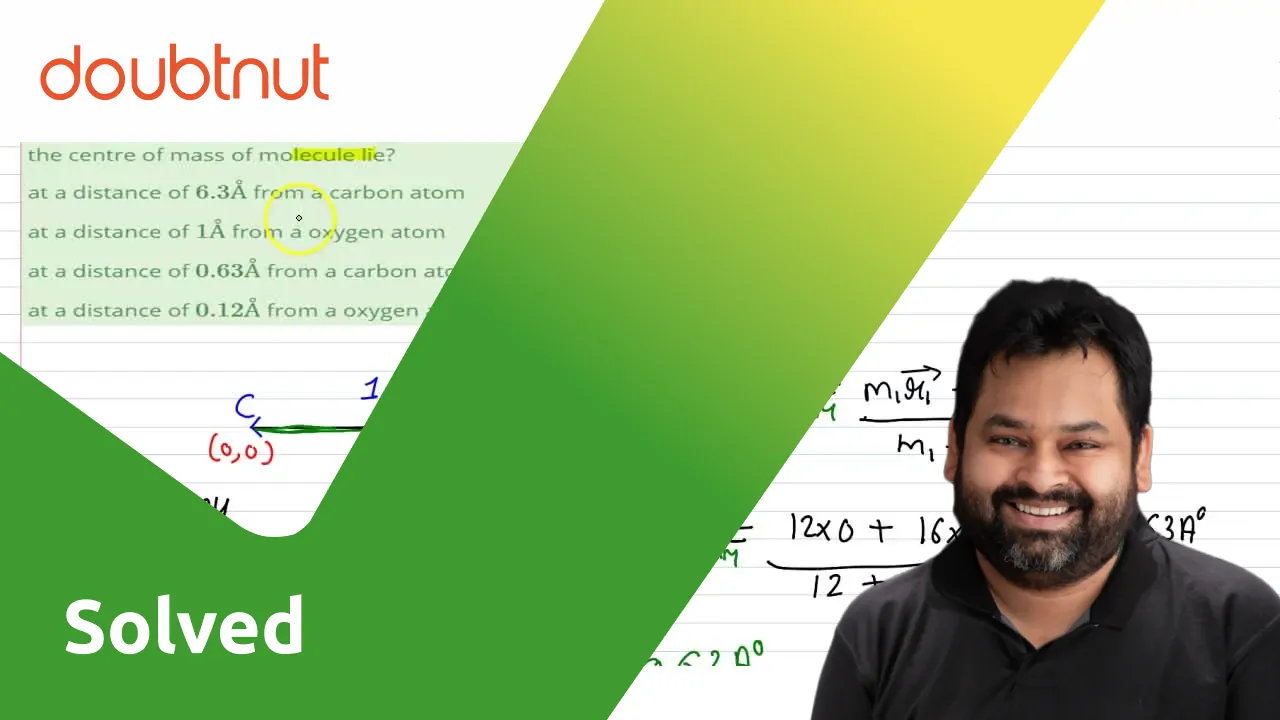Doubtnut App - Get 50 Lakh+ video solutions better than GoogleInstall Now

# In carbon monoxide molecule distance between carbon and oxygen atom is 1.1Å. If mass of carbon atom is 12 amu and oxgyen atom is 16 amu where does the centre of mass of molecule lie? Solution in Gujrati

A

at a distance of 6.3Å from a carbon atom

B

at a distance of 1Å from a oxygen atom

C

at a distance of 0.63Å from a carbon atom

D

at a distance of 0.12Å from a oxygen atom

Text Solution
Verified by Experts

## m1=12,m2=16 →r1=0ˆi+0ˆj,→r2=1.1ˆi+0ˆj →r=m1→r1+m2→r2m1+m2 =16×1.128ˆi=0.63ˆi